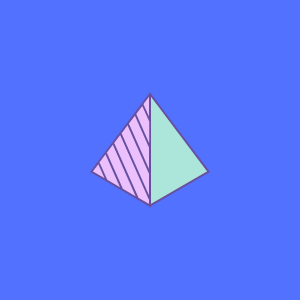# Geometry Symbols

Geometry symbols, and the meaning behind them, can be quite confusing. Who knew that there was so much math behind all of these different figures? Math is an incredibly important piece of geometry, and is essential in understanding it fully.SYMBOL NAME DEFINITION
angle formed by two rays
° degree 1 turn = 360°
deg degree 1 turn = 360deg
Δ triangle triangle shape
prime arcminute, 1° = 60′
π pi constant n/a
double prime arcsecond, 1′ = 60″
AB line segment line from point A to point B
perpendicular perpendicular lines (90° angle)
|x-y| distance distance between points x and y
parallel parallel lines
congruent to equivalence of geometric shapes and size

Geometry symbols, and the meaning behind them, can be quite confusing. Who knew that there was so much math behind all of these different figures? Math is an incredibly important piece of geometry, and is essential in understanding it fully.

Geometry language consists of a set of geometric symbols and rules for their use. Using these symbols allows us to represent lines and curves without using a lot of text or formulas. That makes it more understandable, more convenient and easier.

## Imported Geometry Symbols

That has played a significant role in construction activities since the discovery of the structural strength of concrete and man's imagination to make more beautiful buildings and houses. Construction of bridges, skyscrapers, floors as well as roads takes great use of it.

These symbols are used to create geometrical objects such as lines, circles, rectangles etc.

## Rectangle Symbol

The rectangle has two pairs of parallel sides with rectangles and rhomboids. On each pair, the shorter is called a cathetus and the longer is called an axis.

The line connecting the midpoints of the two sides of a rectangle is called its median, and two lines each parallel to a side and connecting the midpoints of the two sides are called its medial axes.

## Rectangle Types

The rectangle shape is perhaps the most commonly used in geometry and it can be found in various forms throughout history as well as everyday life.

The rectangle is an important shape because it is a principal component of many other geometric figures and can be found within polygons, polyhedra, curves and curves.

### The Square

The square is a basic shape and a quadrilateral with 4 sides and 4 right angles. It can be seen as the 2-D form of many other geometric entities such as the rectangle, rhombus and cuboid. It has many special properties relating to its symmetry, translation and rotational behavior. Squares are pervasive in our daily lives owing to their practical usefulness: only 3 squares are needed to make a house.

### The Kite

A kite is a side view of an (incomplete) Archimedean solid. In geometry, the kite shape is classified as a flabellate. A Flabellate is a polyhedron that has at least one flat face and at least three faces overlapping all others at every vertex other than the two end ones.

### The Parallelogram

A parallelogram in geometry is a polygon with opposite sides that are parallel and adjacent sides that are equal. These properties define the shape, which is also sometimes called a rectangle-trapezoid. A parallelogram does not have to be a rectangle or a trapezoid -- it can have any four congruent sides.

### The Rhombus

In geometry, a rhombus is a parallelogram with all four sides of equal length. The rhombus is diamond-shaped, and its sides form two adjacent right angles.

The word rhombus also applies to a square figure with all sides of equal length and parallel opposite edges: this type of rhombus is often called a diamond square.

### The Trapezoid

A trapezoid is a four-sided plane figure with two pairs of parallel opposite sides. The line that joins the non-parallel sides is called the base.

The two pairs of opposite sides are called the bases and the other two sides are called the legs or the top. A trapezoid has a pair of base angles that add up to 90 degrees and a pair of leg angles that also add up to 90 degrees.

## Degree Symbol

A degree symbol in geometry is a standard mathematical notation that denotes a precise measurement of the angle between two lines. The degree symbol is the letter "°".

The degree symbol can usually be found on the at symbol key of your keyboard. On some keyboards, the degree symbol and the at symbol might be merged into one key. You can also access the degree symbol through your word processing or other applications.